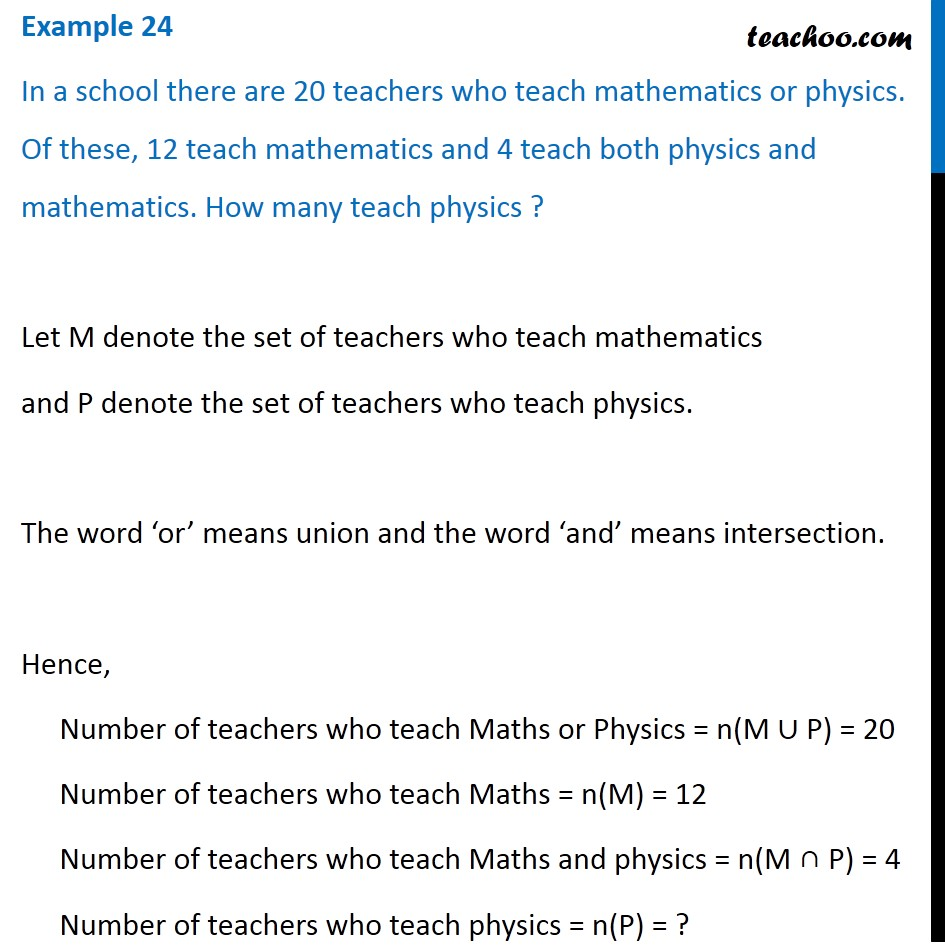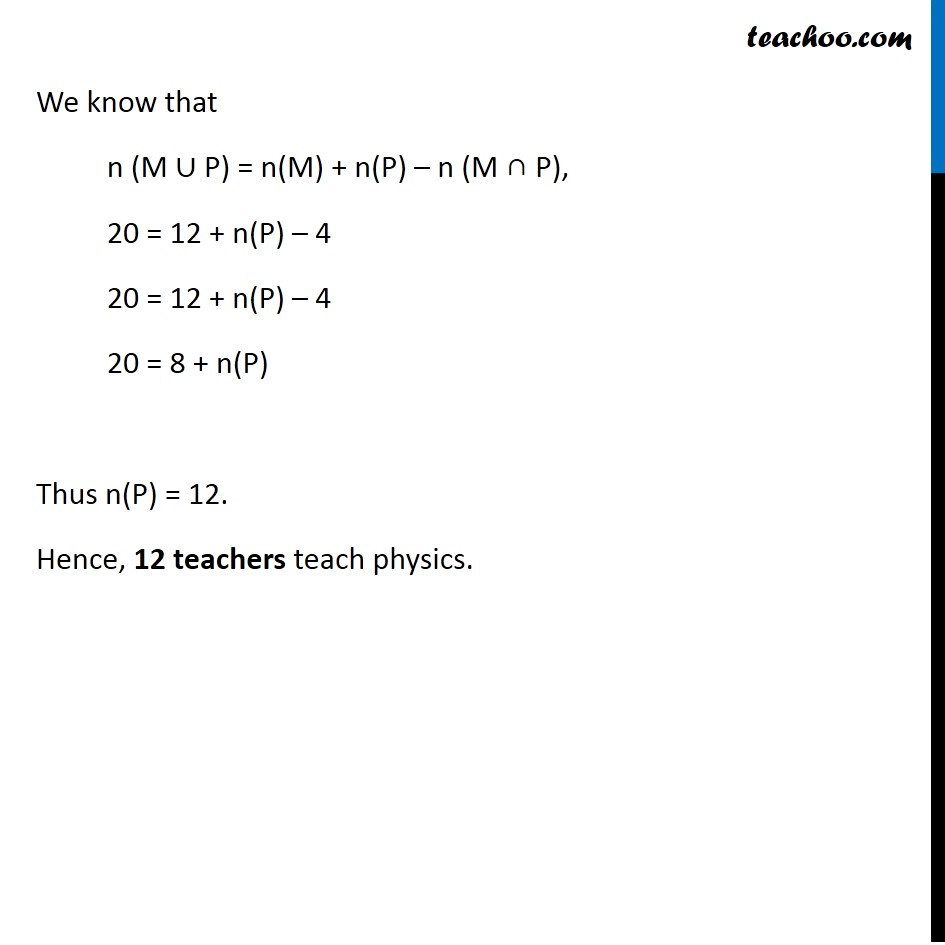1. Chapter 1 Class 11 Sets
2. Concept wise
3. Number of elements in set - 2 sets (Direct)

Transcript

Example 24 In a school there are 20 teachers who teach mathematics or physics. Of these, 12 teach mathematics and 4 teach both physics and mathematics. How many teach physics ? Let M denote the set of teachers who teach mathematics and P denote the set of teachers who teach physics. The word ‘or’ means union and the word ‘and’ means intersection. Hence, Number of teachers who teach Maths or Physics = n(M ∪ P) = 20 Number of teachers who teach Maths = n(M) = 12 Number of teachers who teach Maths and physics = n(M ∩ P) = 4 Number of teachers who teach physics = n(P) = ? We know that n (M ∪ P) = n(M) + n(P) – n (M ∩ P), 20 = 12 + n(P) – 4 20 = 12 + n(P) – 4 20 = 8 + n(P) Thus n(P) = 12. Hence, 12 teachers teach physics.

Number of elements in set - 2 sets (Direct)